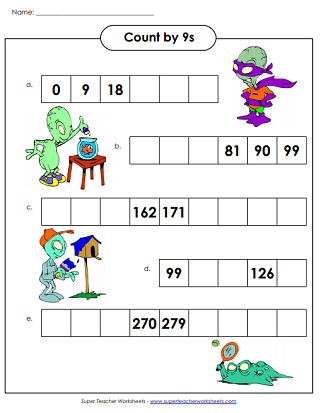# Skip Counting By 9s

These printable worksheets can be used to help your students learn to count by 9s. Count 9, 18, 36, 45, 54, 63, 72, 81, 90, and so on.On this skip-counting worksheet, count by 9s along the number line to find the missing numbers.
Students will fill out all the sections of the caterpillar as they skip count by 9s.
Each penguin has a multiple of 9 written on its tummy. Can you fill in the missing numbers?
Practice skip counting by 9s, then answer the word problems and questions that follow.
Print out this worksheet so your students can practice skip counting by 9s.
More Skip Counting Worksheets

Practice counting by 2s, 3s, 4s, 5s, 6s, 7s, 8s, 10s, 11s, 12s, 25s, and 100s.

Basic Multiplication Worksheets

Super Teacher Worksheets has lots of worksheets for teaching simple multiplication facts.

## Sample Worksheet PicturesMy Account
Site Information